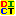DICT.TW Dictionary Taiwan
18.207.133.27

Search for:
[Show options]
[Pronunciation] [Help] [Database Info] [Server Info]

6 definitions found

ra·tio /ˈre(ˌ)ʃo, ʃiˌo/
比率,比例,係數

ra·tio /ˈre(ˌ)ʃo, ʃɪˌo/ 名詞
比,比率,比例

ratio
比,比率 R

From: Network Terminology

ratio
比 比率

Ra·ti·o n.
1. Math. The relation which one quantity or magnitude has to another of the same kind. It is expressed by the quotient of the division of the first by the second; thus, the ratio of 3 to 6 is expressed by or ½; of a to b by a/b; or (less commonly) the second term is made the dividend; as, a:b = b/a.
Note:Some writers consider ratio as the quotient itself, making ratio equivalent to a number.
The term ratio is also sometimes applied to the difference of two quantities as well as to their quotient, in which case the former is called arithmetical ratio, the latter, geometrical ratio. The name ratio is sometimes given to the rule of three in arithmetic. See under Rule.
2. Hence, fixed relation of number, quantity, or degree; rate; proportion; as, the ratio of representation in Congress.
Compound ratio, Duplicate ratio, Inverse ratio, etc. See under Compound, Duplicate, etc.
Ratio of a geometrical progression, the constant quantity by which each term is multiplied to produce the succeeding one.

From: WordNet (r) 2.0